## Summary

They address this problem of domain shift by learning a domain-to-domain image translation GAN to shrink the gap between real and synthetic images. To prove the effectiveness of there method, they train CNN to semantic segmentation on synthetic GTA dataset adapted.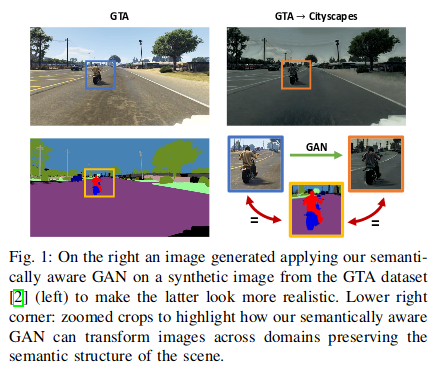## The Method

They have $$X_s$$, $$Y_s$$ the provided source data and associated semantic labels while as $$X_t$$ the provided target data, but without any available target labels. The primary goal of that method is to transform source images (GTA) so to resemble target images (CityScape) while maintaining the semantic content of the scene during the generation process.

### Architecture

The architecture, inspired by the CycleGan1, composed of two generators and two semantic discriminators. The first generator ($$G_{S\rightarrow T}$$), introduce a mapping from source to target domain and produces target samples which should deceive the discriminator ($$D_T$$). $$D_T$$ learns to distinguish between adapted source and true target samples. The second generator ($$G_{T\rightarrow S}$$) learns the opposite mapping from source to target data, and the second discriminator ($$D_S$$) distinguish between adapted target and true source samples. To be able to do semantic segmentation, they add a second decoder to $$D_T$$ and $$D_S$$ obtaining $$D_{S_{sem}}$$ and $$D_{T_{sem}}$$.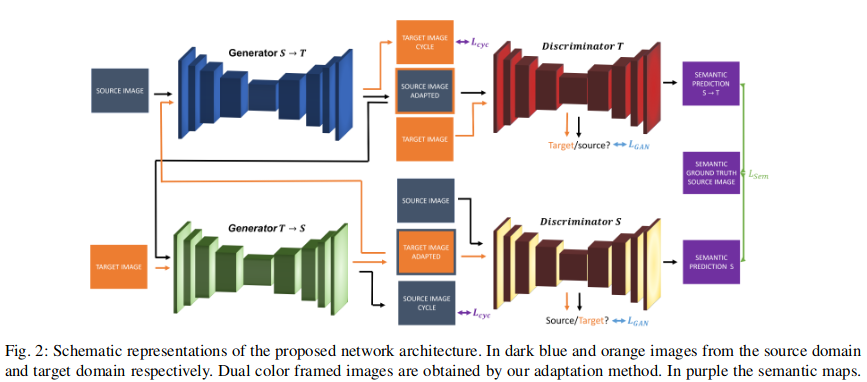### Loss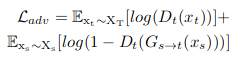#### Semantic Discriminator Loss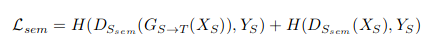#### Weighted Reconstruction Loss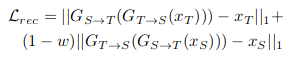#### Final Loss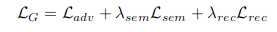## Results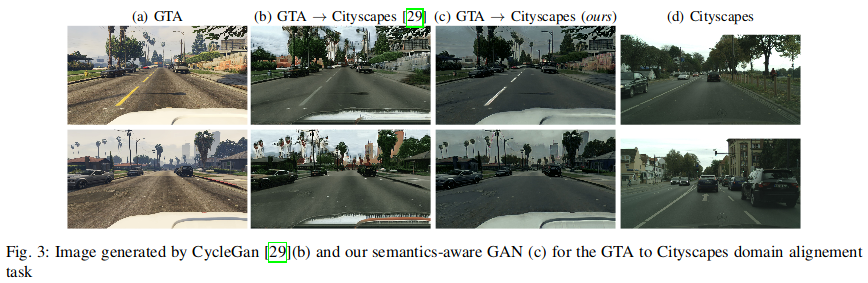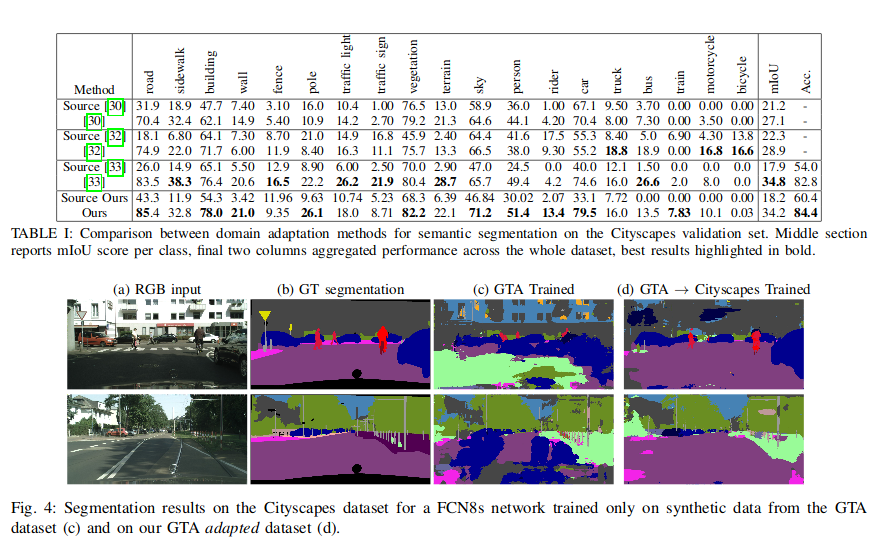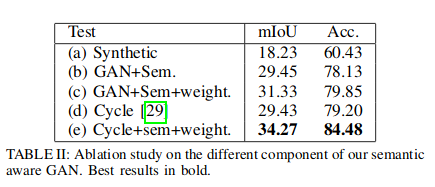1. J.-Y. Zhu, T. Park, P. Isola, and A. A. Efros, “Unpaired image-to-image translation using cycle-consistent adversarial networks,” in The IEEE International Conference on Computer Vision (ICCV), Oct 2017